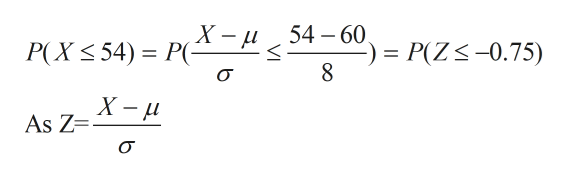A normal distribution has a mean of 60 with a standard deviation of 8. If one score is randomly selected from this distribution, what is the probability that the score will be greater than 54?

Question

A normal distribution has a mean of 60 with a standard deviation of 8. If one score is randomly selected from this distribution, what is the probability that the score will be greater than 54?

Step 1

Let A(x) be the probability that the value of a random variable X is less than or equal to x where X is a random score selected from normal distribution.

Mathematically,

Step 2

Therefore, probability that the score will be greater than 54 will be

Step 3

P(X<=54) can be calculated in ...help_outlineImage Transcriptionclose54 60 _ P(Z-0.75) P(X< 54)= P(- fi = 8 X - Lu As Z - VI fullscreen

Want to see the full answer?

See Solution

Want to see this answer and more?

Our solutions are written by experts, many with advanced degrees, and available 24/7

See Solution
Tagged in

Measures